# Doing data science => Grupo Bimbo deliveries

## September 15, 2016

### Summary

Learn how to use R to explore medium-big, real-world data sets, and how to train an XGBoost model to predict Grupo Bimbo’s sales volume for individual customers.

The problem

I've been ramping up my machine learning skills and competing in competitions, one of which was the Grupo Bimbo competition on Kaggle.com that recently ended. I thought I'd share a summary of the competition and one solution.

## The problem

Grupo Bimbo delivers many products to a plethora of stores around Mexico. Bimbo will take returns from stores when a product has expired, ostensibly to protect their brand reputation. Ideally, Bimbo doesn't want to take any returns, and would like to deliver the exact amount of product that each store needs on a weekly basis.

The main goal of the competition is to predict what the demand for certain products will be for various clients (grocery stores/shops) in Mexico. The data provided consists of 7 weeks worth of orders and returns (labeled as weeks 3-9) from the stores that Bimbo serves in Mexico. The competition is scored based on 2 weeks of data, where the actual demand is hidden (labeled weeks 10 and 11) and used to score participants in the competition.

## Preliminary analyses

First, let's take a look at the data in general. We need to load some libraries/packages before going further:

``````library(data.table)
library(ggplot2)
library(treemap)
library(dplyr) # seems to be necessary for %>% operator for me
library(xgboost)
``````

I was running some of these scripts on an AWS server, and some on my laptop, so I created this small snippet to find the correct path:

``````paths = c("/home/ubuntu/kaggle/bimbo/input/", "/media/nate/Windows/kaggle/bimbo/input/")

for (p in paths){
if (file.exists(paste(p, 'train.csv', sep=''))){
print(paste('path is', p))
break
}
}
``````
``````##  "path is /home/ubuntu/kaggle/bimbo/input/"
``````

Using the `data.table` package, we can read csv files much faster than with `readr`'s `read_csv()` or other csv reading functions in R:

``````train <- fread(paste0(p, 'train.csv'))
``````

The actual week number appears to be well distributed:

``````# plot distribution of weeks - we can see the distribution of weeks is roughly equal
ggplot(train %>% sample_frac(0.1)) +
geom_histogram(aes(x = Semana), color = "black", fill = "red", alpha = 0.5)+
scale_x_continuous(breaks = 1:10)+
scale_y_continuous(name = "Client / Product deliveries")+
theme_bw()
``````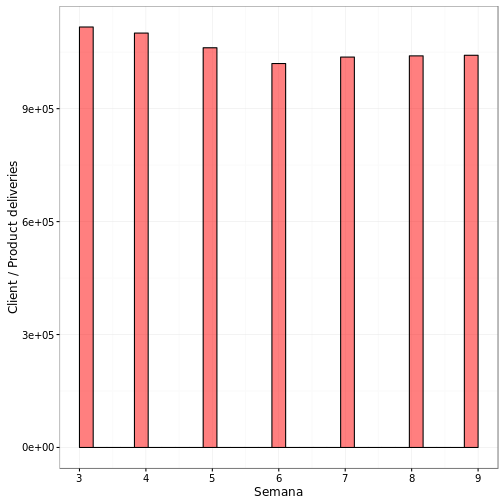However, one thing I discovered is that most (over 50%) of the client/product combinations only constitute 1 or 2 weeks worth of data. In the machine learning section, I assumed these blank weeks were just the mean of the available data for each client/product combo.

``````# this line takes a long, long time to run.  So much for data.table being fast!
# perhaps it's an issue with the uniqueN() function,
# which was a relatively recent addition to data.table I believe
cli_prod_weeks <- train[, .(uniqueWeeks = uniqueN(Semana)), by = .(Cliente_ID, Producto_ID)]

ggplot(cli_prod_weeks) +
geom_histogram(aes(x = uniqueWeeks), color = "black", fill = "red", alpha = 0.5)+
scale_x_continuous(breaks = 1:10)+
scale_y_continuous(name = "Frequency")+
theme_bw()
``````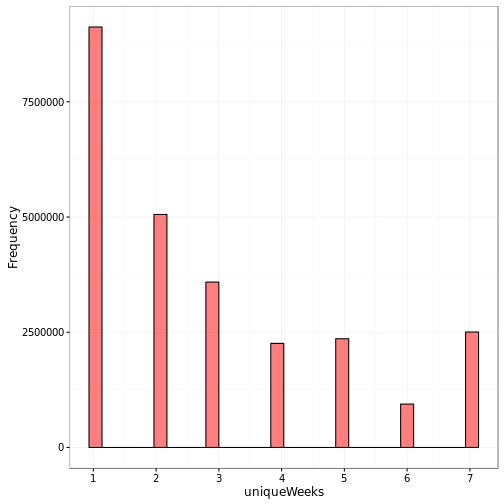``````numUniqueWeeks <- cli_prod_weeks[, .(frequency = .N), by = .(uniqueWeeks)]
numUniqueWeeks <- numUniqueWeeks[order(uniqueWeeks)]

ggplot(numUniqueWeeks[order(uniqueWeeks)], aes(x = uniqueWeeks, y = cumsum(frequency) / sum(frequency))) +
geom_area() +
labs(y = 'cumulative %', x = 'number of unique weeks for each Client/Product combo') +
scale_x_continuous(breaks = 1:7)
``````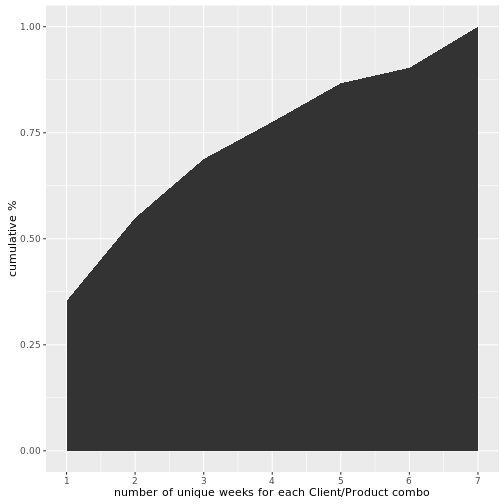We can also see the volume (units delivered each week) for each agency tends to cluster around 10,000.

``````# plot volume by agency
agencias <- train %>%
group_by(Agencia_ID) %>%
summarise(Units = sum(Venta_uni_hoy),
Pesos = sum(Venta_hoy),
Return_Units = sum(Dev_uni_proxima),
Return_Pesos = sum(Dev_proxima),
Net = sum(Demanda_uni_equil)) %>%
mutate(Net_Pesos = Pesos - Return_Pesos,
Return_Rate = Return_Units / (Units+Return_Units)) %>%
arrange(desc(Units)) %>%
inner_join(town, by="Agencia_ID")

ggplot(agencias, aes(x = Units / 7)) +
geom_histogram(fill = "red", color = "gray", binwidth = 10000) +
scale_x_continuous(name = "Units / Week", labels = function(x) paste(x / 1000, "k")) +
scale_y_continuous(name = "Agencias") +
theme_bw()
``````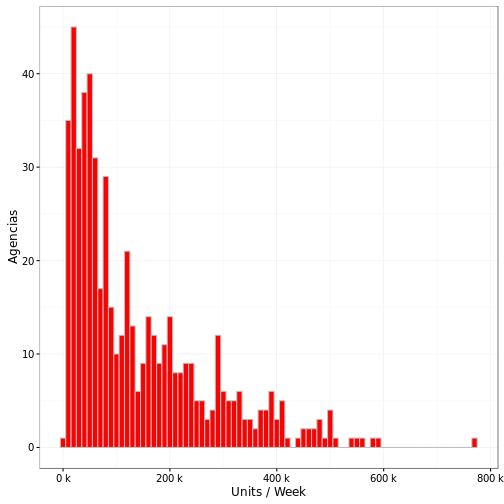Puebla Remision is the client with the largest volume by far, with a few more clients trailing nearby. No one is really sure what Puebla Remision really is, but it doesn't seem to be a normal client, and is probably some sort of internal code of Bimbo's.

``````# top 100 clients treemap
sales <- train %>%
group_by(Cliente_ID) %>%
summarise(Units = sum(Venta_uni_hoy),
Pesos = sum(Venta_hoy),
Return_Units = sum(Dev_uni_proxima),
Return_Pesos = sum(Dev_proxima),
Net = sum(Demanda_uni_equil)) %>%
mutate(Return_Rate = Return_Units / (Units+Return_Units),
Avg_Pesos = Pesos / Units) %>%
mutate(Net_Pesos = Pesos - Return_Pesos) %>%
inner_join(client, by = "Cliente_ID") %>%
arrange(desc(Pesos))

treemap(sales[1:100, ],
index = c("NombreCliente"), vSize = "Units", vColor = "Return_Rate",
palette = c("#FFFFFF","#FFFFFF","#FF0000"),
type = "value", title.legend = "Units return %", title = "Top 100 clients by unit volume",
force.print.labels = T)
``````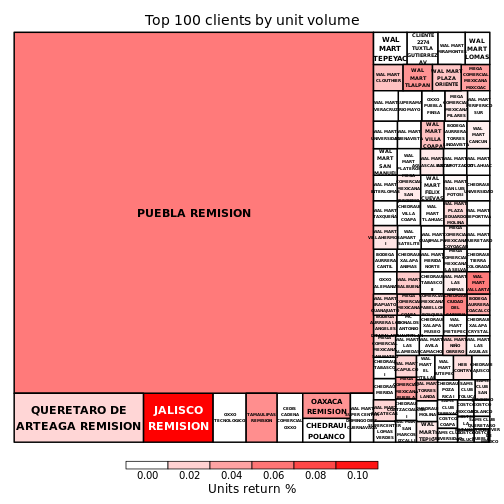Seven of the top 10 clients have 'remision' in their name. Removing clients with 'remision' leaves us with a distribution of clients like this:

``````sales <- as.data.table(sales)
salesNoRem <- sales[!(grepl("REMISION", NombreCliente, ignore.case = T))]

treemap(salesNoRem[1:100, ],
index=c("NombreCliente"), vSize = "Units", vColor = "Return_Rate",
palette = c("#FFFFFF","#FFFFFF","#FF0000"),
type = "value", title.legend = "Units return %", title = "Top 100 clients by unit volume, 'remision's removed",
force.print.labels = T)
``````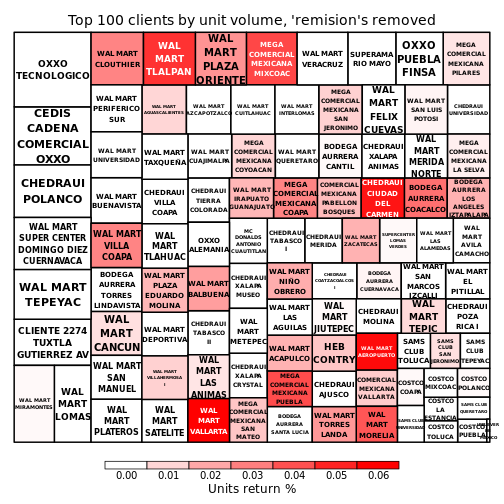Looks like Walmart took over Mexico! The top 100 clients by Peso volume also is dominated by Walmart, although other contributors pop in, like Costco, Sams Club (owned by Walmart), and Superama (also owned by Walmart) appear frequently in the top 100.

While Walmart stores only make up about 0.035 % of the total clients in this database, they represent about 4% of the sales volume by Pesos, and 2% of the sales volume by units.

``````walmarts = sales[grepl('WAL MART|SUPERAMA|SAMS CLUB', NombreCliente, ignore.case = T)]

print(paste('% of clients owned by Walmart:', round(dim(walmarts)/dim(sales)*100, 3)))
``````
``````##  "% of clients owned by Walmart: 0.036"
``````
``````print(paste('% of Pesos volume from Walmart:', round(sum(walmarts\$Pesos)/sum(sales\$Pesos)*100), 2))
``````
``````##  "% of Pesos volume from Walmart: 4 2"
``````
``````print(paste('% of Units volume from Walmart:', round(sum(walmarts\$Units)/sum(sales\$Units)*100, 2)))
``````
``````##  "% of Units volume from Walmart: 1.94"
``````
``````treemap(salesNoRem[1:100, ],
index = c("NombreCliente"), vSize = "Pesos", vColor = "Return_Rate",
palette = c("#FFFFFF","#FFFFFF","#FF0000"),
type = "value", title.legend = "Units return %", title = "Top 100 clients by Pesos volume, 'remision's removed",
force.print.labels = T)
``````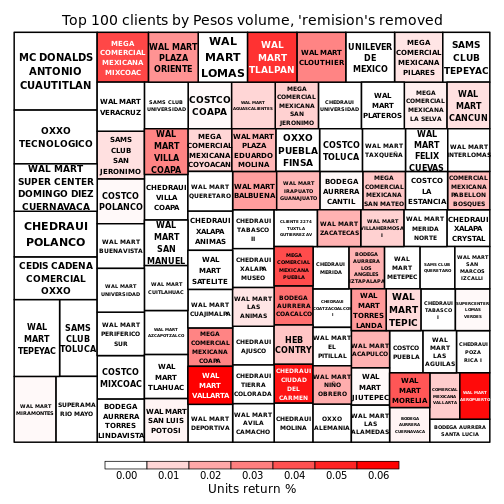We can also take a look at the top agencies and products. The top products are mostly small packages (under 200g) of dessert cakes, like the twinkies of Mexico.

``````# plot top 100 products in treemap
products <- train %>% group_by(Producto_ID) %>%
summarise(Units = sum(Venta_uni_hoy),
Pesos = sum(Venta_hoy),
Return_Units = sum(Dev_uni_proxima),
Return_Pesos = sum(Dev_proxima),
Net = sum(Demanda_uni_equil)) %>%
mutate(Avg_Pesos = Pesos / Units,
Return_Rate = Return_Units / (Units + Return_Units)) %>%
filter(!is.nan(Avg_Pesos)) %>%
inner_join(prods, by = "Producto_ID") %>%
arrange(desc(Units))

products\$NombreProducto <- factor(as.character(products\$NombreProducto), levels = products\$NombreProducto)

treemap(products[1:100, ],
index = c("NombreProducto"), vSize = "Units", vColor = "Return_Rate",
palette = c("#FFFFFF","#FFFFFF","#FF0000"),
type = "value", title.legend = "Units return %", title = "Top 100 products")
``````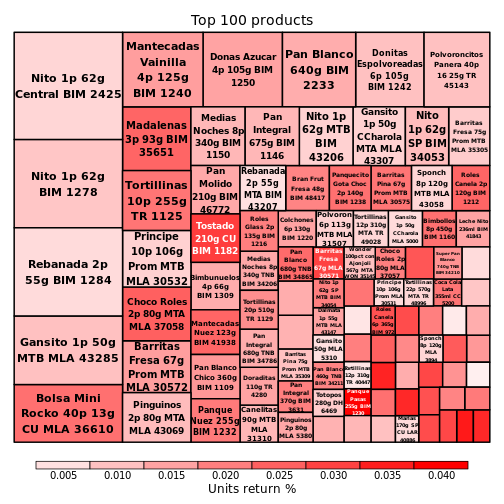``````# number of products per client
products.by.client <- train %>%
group_by(Cliente_ID) %>%
summarise(n_products = n_distinct(Producto_ID)) %>%
inner_join(client, by = "Cliente_ID")

ggplot(products.by.client) +
geom_histogram(aes(x = n_products), fill = "red", color = "black", alpha = "0.3", binwidth = 2)+
scale_x_continuous(name = "Number of products by client", lim = c(0, 150)) +
scale_y_continuous(name = "Number of clients", labels = function(x) paste(x / 1000, "k")) +
theme_bw()
``````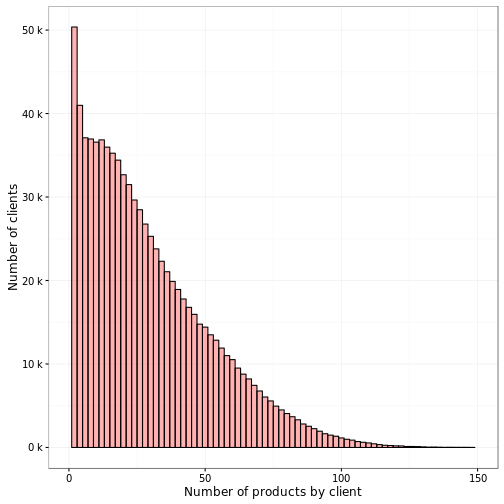``````products.by.client <- as.data.table(products.by.client)
walmart.products <- products.by.client[grepl('WAL MART|SUPERAMA|SAMS CLUB', NombreCliente, ignore.case = T)]

ggplot(walmart.products) +
geom_histogram(aes(x = n_products), fill = "red", color = "black", alpha = "0.3", binwidth = 2)+
scale_x_continuous(name = "Number of products by client", lim = c(0, 150)) +
scale_y_continuous(name = "Number of clients", labels = function(x) paste(x / 1000, "k")) + ggtitle("Number of products for Walmart stores") +
theme_bw()
``````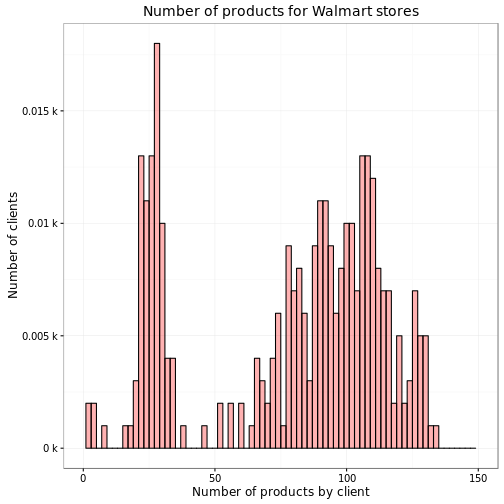Now for the fun part…actually predicting some data. For the xgboost model, we only need some of the columns from train and test. The following code would help reduce memory usage and load time:

``````train <- fread(paste0(p, 'train.csv'), select = c("Semana", 'Cliente_ID', 'Producto_ID', 'Agencia_ID', 'Ruta_SAK', 'Demanda_uni_equil'))
test <- fread(paste0(p, 'test.csv'), select = c("Semana", 'id', 'Cliente_ID', 'Producto_ID', 'Agencia_ID', 'Ruta_SAK'))
``````

One thing I tried was extracting the weights and brands from the product names, but they actually decreased performance of the classifier/prediction, so I didn't use them.

``````# the evaluation of this block was turned off,
# it's just here for reference and doesn't actually do
# anything
library(stringr)
# get weights from products and join into train and test things
# update by reference
prods[, weight := str_extract(NombreProducto, "([0-9]+)[kgKG]+")]
prods[, weight := str_extract(weight, "[0-9]+")]
prods[, weight := as.numeric(weight)]
prods\$weight[is.na(prods\$weight)] <- mean(prods\$weight, na.rm=T)

# extract brand from product name
prods[, brand := str_extract(NombreProducto, "([A-Z]+)[:blank:]+[0-9]+\$")]
prods[, brand := str_extract(brand, "[A-Z]+")]
brands <- factor(prods\$brand)
prodMode <- sort(brands, decreasing = T)
# check index of where the NA is: which(is.na(prods\$brand))
prods\$brand[is.na(prods\$brand)] <- prodMode

# finally, add the brands and weights to the train and test
train <- merge(train, prods, all.x = T, by = "Producto_ID")
test <- merge(test, prods, all.x = T, by = "Producto_ID")
train\$NombreProducto <- NULL
test\$NombreProducto <- NULL
gc()
``````

Next, we combine train and test to make one large dataset:

``````train\$id <- 0
train[, target := Demanda_uni_equil]
train[, Demanda_uni_equil := NULL]
train[, tst := 0]
test\$target = 0
test[,tst := 1]
data <- rbind(train, test)
rm(test)
rm(train)
gc()
``````

Now the most imporant part–creating the timeseries data. We will create l1, l2, l3, l4, and l5 values for the target values (demand) from 1, 2, 3, 4, and 5 weeks prior. The way this works is as follows: 1. create a temporary dataset (data1) where each week is set to be the next week in the future 2. We can only use weeks 8 and 9 in the training data since we are using 5 lagged weeks, and the test data is only weeks 10 and 11. We keep this data from the temp dataset (data1) and merge it with the our main dataset. We need to take the mean of the target for the lagged target variable, because sometimes deliveries were made from different agencies.

To enable this to run on a lower-RAM machine (this barely worked for me on my laptop with 16GB of RAM), we can remove the data we're not using anymore after each merge. These are the `data <- data[Semana > 3]` lines.

The merge step of this process seems to take the longest.

``````# Creating features for one week lagged values of target variable
data1 <- data[, .(Semana = Semana + 5, Cliente_ID, Producto_ID, target)]
data <- merge(data, data1[Semana >= 8 & Semana <= 11, .(targetl5 = mean(target)), by = .(Semana, Cliente_ID, Producto_ID)], all.x = T, by = c("Semana", "Cliente_ID", "Producto_ID"))
data <- data[Semana > 3]

data1 <- data[, .(Semana = Semana + 4, Cliente_ID, Producto_ID, target)]
data <- merge(data, data1[Semana >= 8 & Semana <= 11, .(targetl4 = mean(target)), by = .(Semana, Cliente_ID, Producto_ID)], all.x = T, by = c("Semana", "Cliente_ID", "Producto_ID"))
data <- data[Semana > 4]

data1 <- data[, .(Semana = Semana + 3, Cliente_ID, Producto_ID, target)]
data <- merge(data, data1[Semana >= 8 & Semana <= 11, .(targetl3 = mean(target)), by = .(Semana, Cliente_ID, Producto_ID)], all.x = T, by = c("Semana", "Cliente_ID", "Producto_ID"))
data <- data[Semana > 5]

data1 <- data[, .(Semana = Semana + 2, Cliente_ID, Producto_ID, target)]
data <- merge(data, data1[Semana >= 8 & Semana <= 11, .(targetl2 = mean(target)), by = .(Semana, Cliente_ID, Producto_ID)], all.x = T, by = c("Semana", "Cliente_ID", "Producto_ID"))
data <- data[Semana > 6]

data1 <- data[, .(Semana = Semana + 1, Cliente_ID, Producto_ID, target)]
data <- merge(data, data1[Semana >= 8 & Semana <= 11, .(targetl1 = mean(target)), by = .(Semana, Cliente_ID, Producto_ID)], all.x = T, by = c("Semana", "Cliente_ID", "Producto_ID"))

data <- data[Semana > 7]
rm(data1)
gc() # minimize memory usage
``````

Essentially, after this time series creation, we will be training our XGB classifier on weeks 8 and 9 in the training set, and we will already have our lagged data features created for the test set.

Next we create some measures of frequency for agencies, routes, clients, and products, aveaged over all weeks.

``````# Creating frequency features for some factor variables
nAgencia_ID <- data[, .(nAgencia_ID = .N), by = .(Agencia_ID, Semana)]
nAgencia_ID <- nAgencia_ID[, .(nAgencia_ID = mean(nAgencia_ID, na.rm=T)), by = Agencia_ID]
data <- merge(data, nAgencia_ID, by = 'Agencia_ID', all.x = T)

nRuta_SAK <- data[, .(nRuta_SAK = .N), by = .(Ruta_SAK, Semana)]
nRuta_SAK <- nRuta_SAK[, .(nRuta_SAK = mean(nRuta_SAK, na.rm = T)), by = Ruta_SAK]
data <- merge(data, nRuta_SAK, by = 'Ruta_SAK', all.x = T)

nCliente_ID <- data[, .(nCliente_ID = .N), by = .(Cliente_ID, Semana)]
nCliente_ID <- nCliente_ID[, .(nCliente_ID = mean(nCliente_ID, na.rm = T)), by = Cliente_ID]
data <- merge(data, nCliente_ID, by = 'Cliente_ID', all.x = T)

nProducto_ID <- data[, .(nProducto_ID = .N), by = .(Producto_ID, Semana)]
nProducto_ID <- nProducto_ID[, .(nProducto_ID = mean(nProducto_ID, na.rm = T)), by = Producto_ID]
data <- merge(data, nProducto_ID, by = 'Producto_ID', all.x = T)
``````

Let's actually check out some of these frequencies… Agencies and routes are dominated by a few, while the clients are dominated even more strongly by a few. This is the 'Puebla Remision' we saw earlier. Products are also strongly dominated by a few. The Cliente ID frequency has one client with a very large frequency (~12,000), this is the 'PUEBLA REMISION' we saw earlier. Seven of the top ten clients by frequency are some sort of 'remision'.

``````ggplot(nAgencia_ID, aes(x = nAgencia_ID)) + geom_histogram()
``````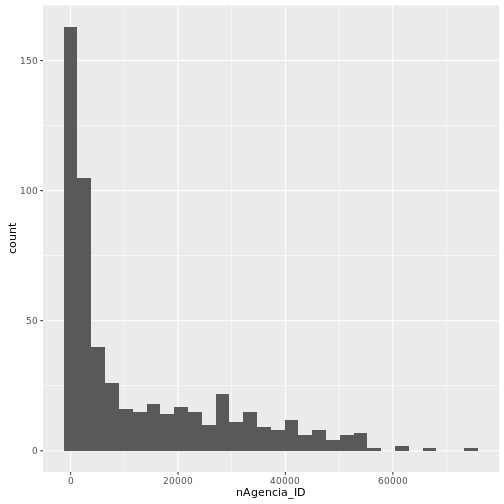``````ggplot(nRuta_SAK, aes(x = nRuta_SAK)) + geom_histogram()
``````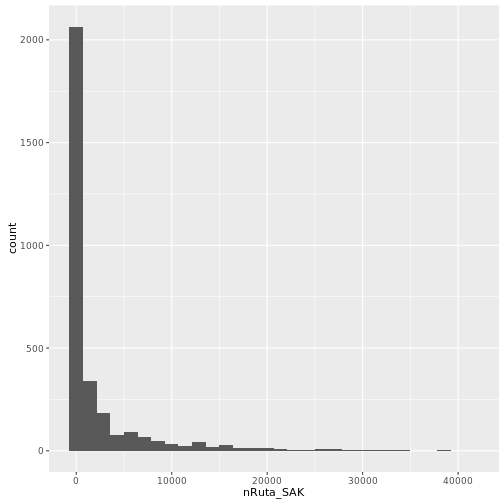``````ggplot(nCliente_ID, aes(x = nCliente_ID)) + geom_histogram()
``````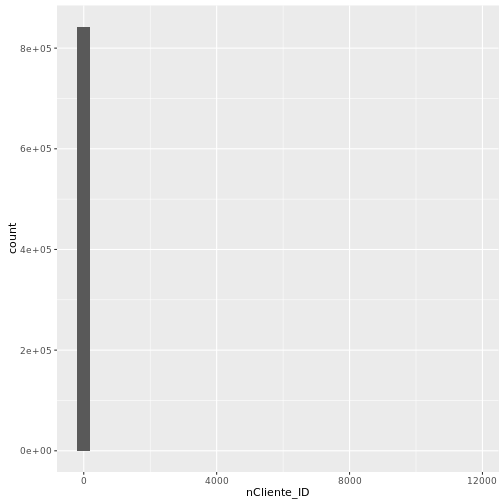``````print(paste("mean cliente ID frequency: ", mean(nCliente_ID\$nCliente_ID)))
print(paste("max cliente ID frequency: ", max(nCliente_ID\$nCliente_ID)))

# remove the 'remision's again to see the actual distribution of client frequencies

top_clients <- merge(nCliente_ID, client, by = "Cliente_ID", all.x = T)
top_clients <- top_clients[!(grepl("REMISION", NombreCliente, ignore.case = T))]
top_clients <- top_clients[order(-nCliente_ID)]
ggplot(top_clients, aes(x = nCliente_ID)) +
geom_histogram() +
ggtitle("client frequency with 'remision's removed")
``````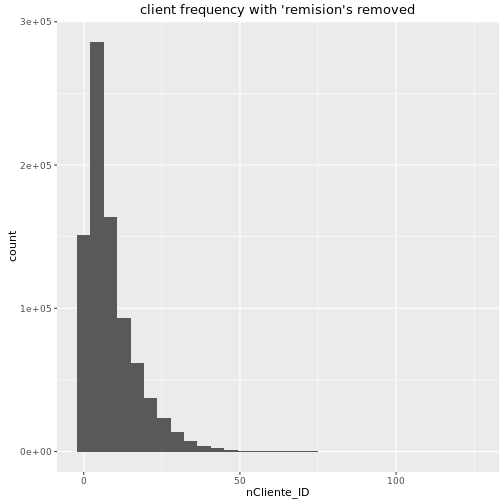``````# we don't need this data any more so we'll remove it to conserve memory
rm(nAgencia_ID)
rm(nRuta_SAK)
rm(nCliente_ID)
rm(top_clients)
rm(nProducto_ID)
gc()
``````

Next, we transform the demand by taking the natural logarithm. We have to add 1 to make sure we don't get negative infinity for 0 demand. Looking at the histogram of demands, we can see that there are a few data points with large orders. We take the natural log to help normalize these outliers.

``````ggplot(data, aes(x = target)) + geom_histogram() +
scale_y_log10(name = 'counts') +
ggtitle("demand target before scaling with log")
``````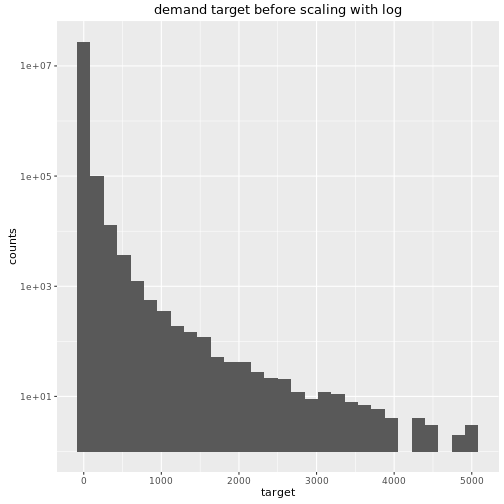``````# make train and test datasets
data\$target <- log(data\$target + 1)
data_train <- data[tst == 0,]
data_test <- data[tst == 1,]
``````

Now looking at the histogram after log-transforming the target, we can see the data is more tightly distributed.

``````ggplot(data, aes(x = target)) + geom_histogram() +
scale_y_log10(name = 'log(counts)') +
ggtitle("demand target after scaling with log")
``````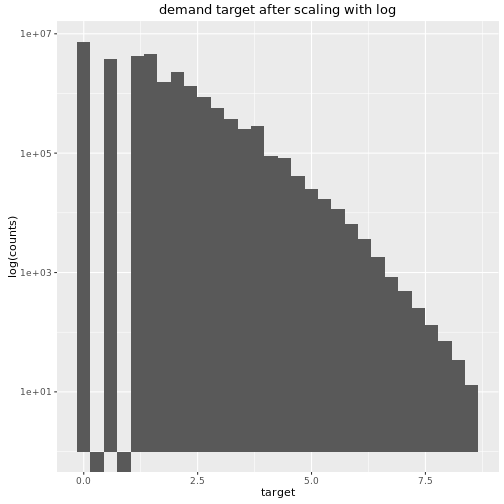``````# we don't need the data data.table anymore
rm(data)
gc()
``````
``````# I dynamically generated my filenames to keep track of which model perfomed in which way.
# On my ubuntu server, I would run something like:
# Rscript --verbose XGB-aa14.R > output-aa14.out
# changing the name of aa14 to whatever version I was running at the time
fVersName <- 'aa14'

# create a list of features for creating the xgb matrix
features <- names(data_train)[!(names(data_train) %in% c('id', 'target', 'tst'))]

# always make sure to set the seed when doing something with random
# generation if you want the results to be reproducible
set.seed(42)

# create a random sample of the train data for live evaluation of the XGB
# classifier
wltst <- sample(nrow(data_train), 30000)
# create the special xgb matrix for the model
dval <- xgb.DMatrix(data = data.matrix(data_train[wltst, features,with = F]),
label = data.matrix(data_train[wltst, target]), missing = NA)
watchlist <- list(dval = dval)

# this is an important step--setting the parameters
# These are the best parameters I've found
# for a short runtime
params <- list(objective = "reg:linear",
booster = "gbtree",
eta = 0.1,
max_depth = 10,
subsample = 0.5,
colsample_bytree = 0.7)

nrounds <- 100
# make xgb training matrix
data <- xgb.DMatrix(data = data.matrix(data_train[-wltst, features, with=F]), label=data.matrix(data_train[-wltst, target]), missing = NA)

# create and train the XGBoost classifier
clf <- xgb.train(params = params,
data = data,
nrounds = nrounds,
verbose = 1,
print.every.n = 10,
watchlist = watchlist,
maximize = F,
eval_metric = 'rmse')
``````
``````##   dval-rmse:1.263519
##  dval-rmse:0.644289
##  dval-rmse:0.506942
##  dval-rmse:0.479168
##  dval-rmse:0.469776
##  dval-rmse:0.465876
##  dval-rmse:0.463074
##  dval-rmse:0.461002
##  dval-rmse:0.459639
##  dval-rmse:0.458401
``````
``````# Make prediction for the 10th week
data_test1 <- data_test[Semana == 10,]
pred <- predict(clf, xgb.DMatrix(data.matrix(data_test1[, features, with = F]), missing = NA))
# we need to exponentiate our data to bring it back to it's normal form
res <- exp(round(pred, 5)) - 1

# Create lagged values of target variable which will be used as a feature for the 11th week prediction
data_test_lag1 <- data_test1[, .(Cliente_ID, Producto_ID)]
data_test_lag1\$targetl1 <- res
data_test_lag1 <- data_test_lag1[, .(targetl1 = mean(targetl1)), by = .(Cliente_ID, Producto_ID)]

results10 <- data.frame(id = data_test1\$id, Demanda_uni_equil = res)

data_test2 <- data_test[Semana == 11,]
data_test2[, targetl1 := NULL]

# Merge lagged values of target variable to test the set for the 11th week
data_test2 <- merge(data_test2, data_test_lag1, all.x = T, by = c('Cliente_ID', 'Producto_ID'))
pred <- predict(clf, xgb.DMatrix(data.matrix(data_test2[, features,with = F]), missing = NA))
res <- exp(round(pred, 5)) - 1
results11 <- data.frame(id = data_test2\$id, Demanda_uni_equil = res)
results <- rbind(results10, results11)

# any results predicted to be less than 0 should just be 0
results[results[, 2] < 0, 2] <- 0
results[, 2] <- round(results[, 2], 1) # demand for items should be an integer
results[, 1] <- as.integer(results[, 1]) #
class(results[,1]) <- 'int32'
options(digits = 18)
write.csv(results, file = paste0('/home/ubuntu/kaggle/bimbo/submissions/R-XGB-5daylag-', fVersName, '.csv'), row.names = F)
``````

And there we have it, this got me a score of 0.48850, about 600th (top ~30%) in the competition. This particular script will get you about 0.48888 on the leaderboard. I actually modified the number of trees (nrounds) to be 5000 and the learning rate (eta) to be small (0.01) to get the best score, and the lookback creation was slightly different–I was only using weeks greater than 8 in the training. The main beef of the machine learning was adapted from a script Bohdan Pavlyshenko, who was a member of the winning team of the competition. Some of the exploratory analysis was adapted from this very nice report here.

If you want to run this script yourself, you'll have to first get the data from the challenge, change the filepath (the 'paths' variable at the top of the page) and the filepath for writing the submissions file at the end.

Updated on

### HPC GPU Cluster

An overview of a HPC GPU cluster I created at Regis University. Continue reading

#### Regis Ransomware

Published on January 16, 2020

#### TensorFlow functions with Keras

Published on February 21, 2019